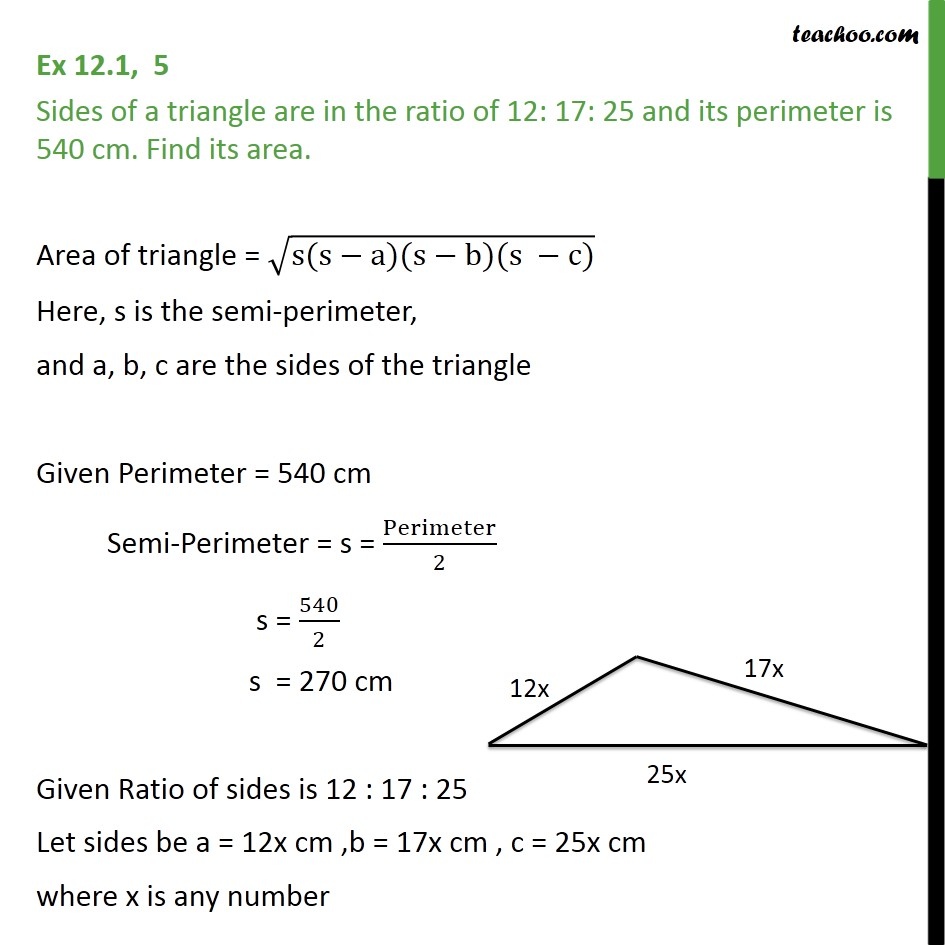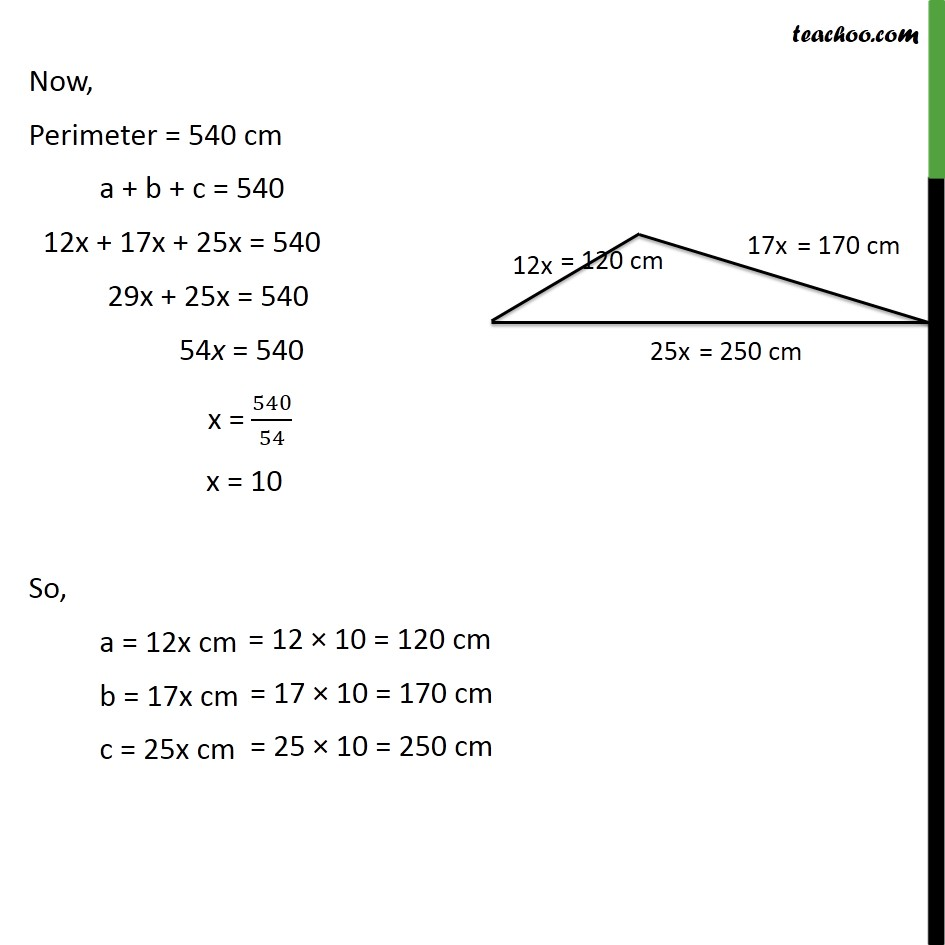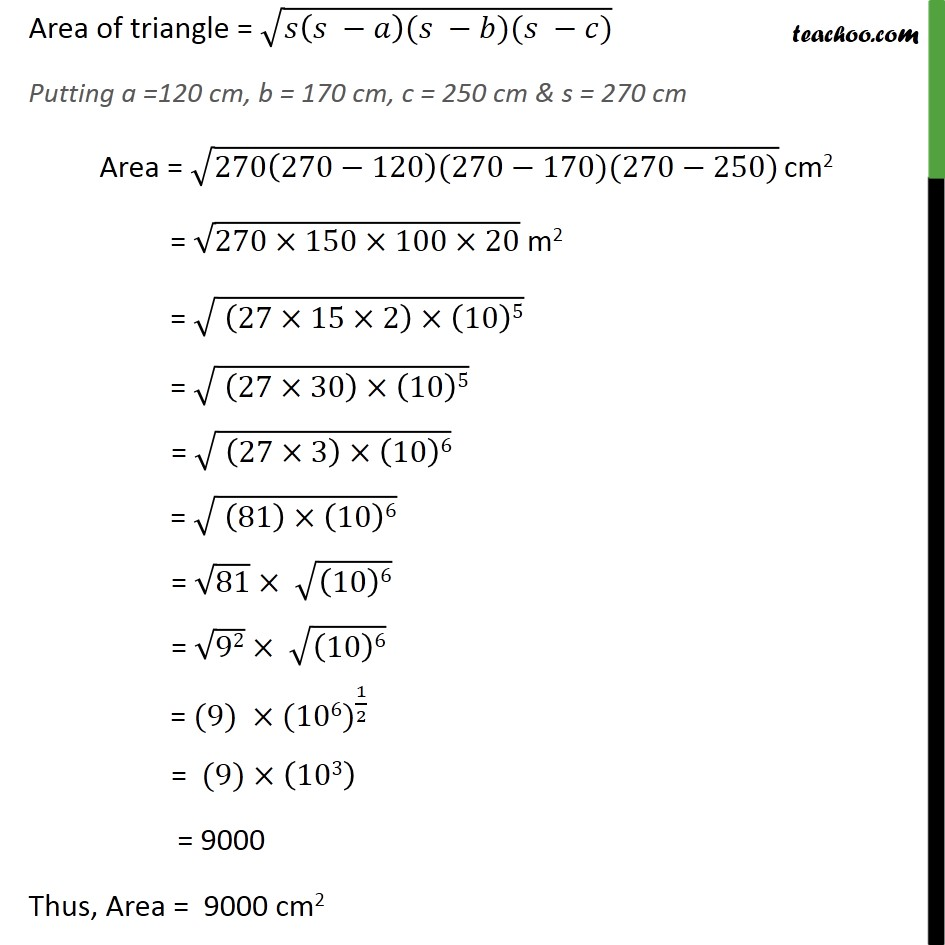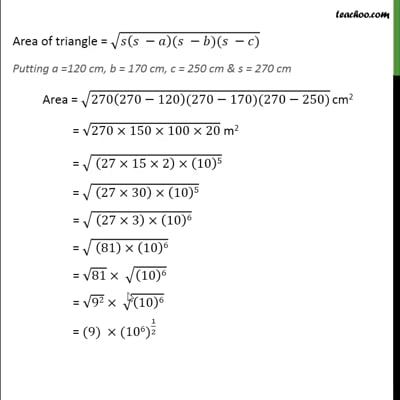Finding area of triangle

Chapter 12 Class 9 Herons Formula
Concept wiseThis video is only available for Teachoo black users

Introducing your new favourite teacher - Teachoo Black, at only ₹83 per month

### Transcript

Ex 12.1, 5 Sides of a triangle are in the ratio of 12: 17: 25 and its perimeter is 540 cm. Find its area. Area of triangle = (s(s a)(s b)(s c)) Here, s is the semi-perimeter, and a, b, c are the sides of the triangle Given Perimeter = 540 cm Semi-Perimeter = s = Perimeter/2 s = 540/2 s = 270 cm Given Ratio of sides is 12 : 17 : 25 Let sides be a = 12x cm ,b = 17x cm , c = 25x cm where x is any number Now, Perimeter = 540 cm a + b + c = 540 12x + 17x + 25x = 540 29x + 25x = 540 54x = 540 x = 540/54 x = 10 So, a = 12x cm b = 17x cm c = 25x cm Area of triangle = ( ( )( )( )) Putting a =120 cm, b = 170 cm, c = 250 cm & s = 270 cm Area = (270(270 120)(270 170)(270 250)) cm2 = (270 150 100 20) m2 = ( (27 15 2) (10)5) = ( (27 30) (10)5) = ( (27 3) (10)6) = ( (81) (10)6) = 81 ((10)6) = 92 ((10)6) = (9) (106) ^(1/2) = (9) (103) = 9000 Thus, Area = 9000 cm2